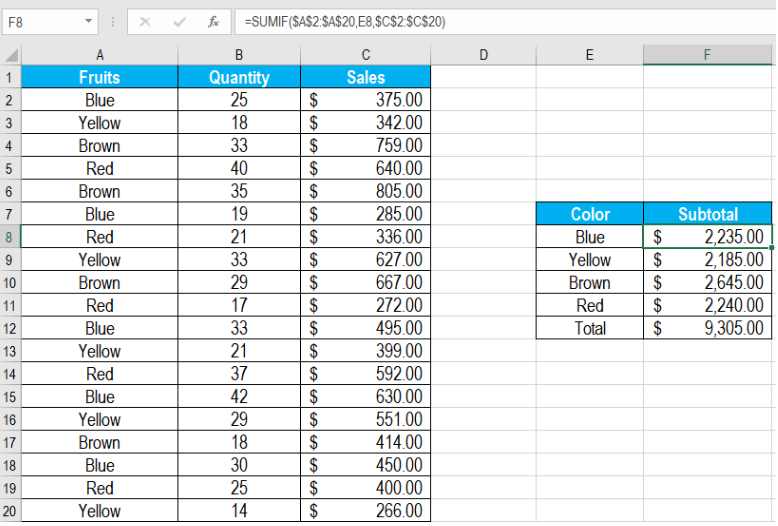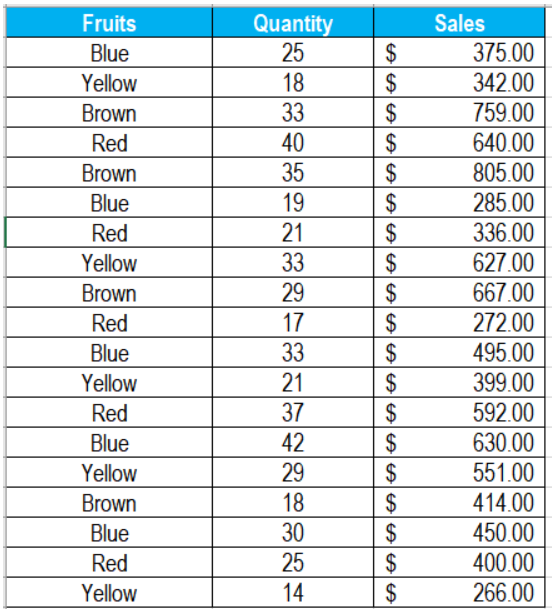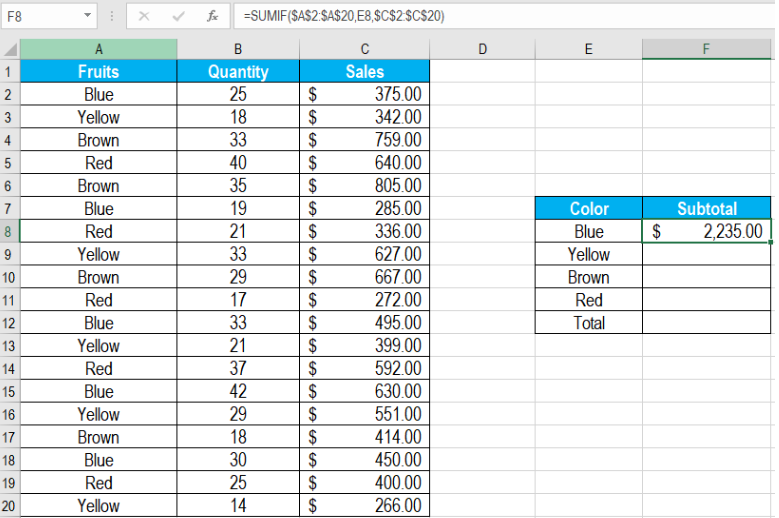Get instant live expert help with Excel or Google Sheets“My Excelchat expert helped me in less than 20 minutes, saving me what would have been 5 hours of work!”

#### Post your problem and you’ll get Expert help in seconds.

Your message must be at least 40 characters
Our professional Expert are available now. Your privacy is guaranteed.

# How to Find Subtotal by Color in Excel

Excel helps us to subtotal by color in an efficient manner. We can use the SUMIF function to perform subtotal by color in Excel. It helps to add cells based on numbers, dates, and text matching certain criteria. In this tutorial, we will learn how to subtotal by color in Excel.Figure 1. Example of How to Subtotal by Color

## Generic Formula

`=SUMIF(color_range,criteria,number_range)`

• range_color: This argument is required. It is the range for which the criteria of color will be applied. This takes an absolute reference and is not changed when we copy the formula down.
• criteria: This is also required. Picks up the criteria from the adjacent cell. Uses a relative reference. Changes when copied down.
• Range_number: This value is optional. The cells which are to be added together by SUMIF.

Here, we apply the criteria to the range of cells provided as range_color. Next, the reference obtains the criteria. Then, range_number specifies the cells that Excel adds together. Finally, subtotal adds the numbers and returns the result.

## Setting Up Data

The following example uses a wall paint sales database for four colors. Column A has the color names, column B the quantity and column C the sales.Figure 2. The Sample Data Set

To find out the subtotal by color in column F:

• We need to go to cell F8.
• Assign the formula `=SUMIF(\$A\$2:\$A\$20,E8,\$C\$2:\$C\$20)` to cell F8.
• Press Enter.Figure 3. Example of Applying the Formula to Subtotal by Color

• Drag the formula from cells F8 to F11 using the fill handle on the bottom right.

This will show the subtotal by colors in column F.

We can subtotal by color using the SUMIF function very easily. However, if we want to  count or add cells filled with colors, we need to do it in a different way. We can use the same logic to count the cells when using conditional formatting to apply colors. If the cells are filled with colors manually, we’ll need to follow a different approach.

Most of the time, the problem you will need to solve will be more complex than a simple application of a formula or function. If you want to save hours of research and frustration, try our live Excelchat service! Our Excel Experts are available 24/7 to answer any Excel question you may have. We guarantee a connection within 30 seconds and a customized solution within 20 minutes.

### Did this post not answer your question? Get a solution from connecting with the expert.Another blog reader asked this question today on Excelchat:
Solution examplesamending a formula so that there is another criteria and if it does not meet this criteria then we stick with the original criteria
Solved by A. B. in 40 minsI need Column D to = Column C - Column A only if Column B has a 'Date' entered.
Solved by I. B. in 11 minsHello, I need help trying to create a sum of numerical values on a spreadsheet in which the only values that apply to the sum are when the status of another cell is a certain value (e.g. value is not in sum when status: closed, value is in sum when status: open)
Solved by T. W. in 18 minsI am looking for a formula to SUM column L, if Column A matched the relevant date and column C matches the name
Solved by Z. Y. in 16 minsI need a formula that is essentially saying IF this range of cells contain a certain name, add the number in another cell on the same row, and them sum that number in a different cell..!
Solved by O. H. in 44 mins## Subscribe to Excelchat.coAnother blog reader asked this question today on Excelchat: